# OECD Net Oda data analysis 8 - PCA(Principal Component Analysis) shows G7 countriesPhoto by Navi Photography on Unsplash

This post is following of above post.

In this post, I use PCA(Principal Component Analysis).
First, I make a data frame for PCA.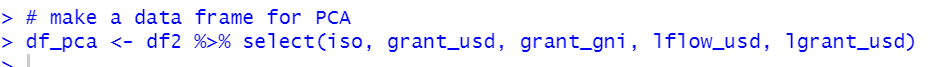Then, I use prcomp() function to do PCA.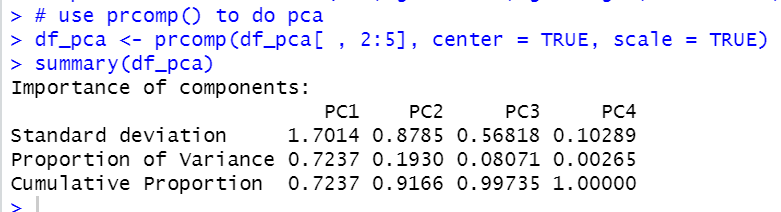We see PC1 explaines 72.4% of data set variance, PC1 and PC2 explaines 91.7% of data set variance.

Let's plot PC1 and PC2.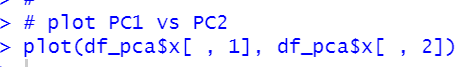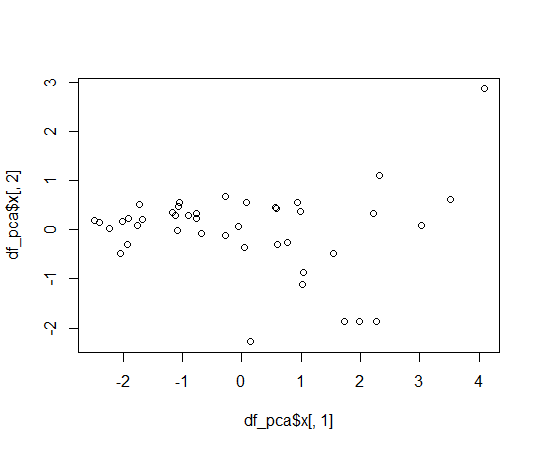Okay, let's plot iso code on the plot.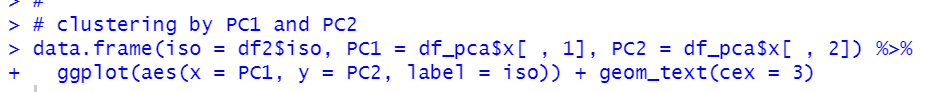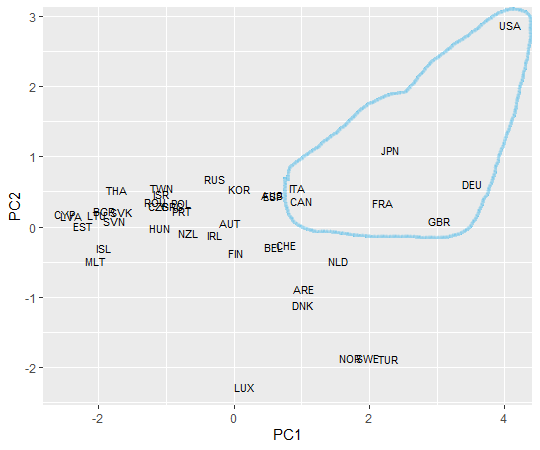We see G7 countries are plotted at upper right hand area.
That' it! Thank you!

For the 1st post,